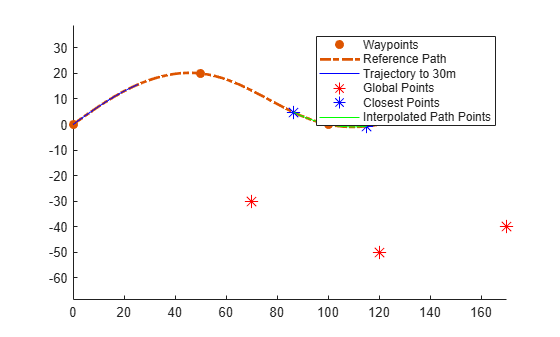# interpolate

Interpolate reference path at provided arc lengths

## Syntax

``pathPoints = interpolate(refPath,arclengths)``

## Description

example

````pathPoints = interpolate(refPath,arclengths)` interpolates the reference path at the provided arc lengths and returns the interpolated points on the path in global coordinates.```

## Examples

collapse all

Generate a reference path from a set of waypoints.

```waypoints = [0 0; 50 20; 100 0; 150 10]; refPath = referencePathFrenet(waypoints);```

Create a trajectoryGeneratorFrenet object from the reference path.

`connector = trajectoryGeneratorFrenet(refPath);`

Generate a five-second trajectory between the path origin and a point 30 m down the path as Frenet states.

```initState = [0 0 0 0 0 0]; % [S dS ddS L dL ddL] termState = [30 0 0 0 0 0]; % [S dS ddS L dL ddL] frenetTraj = connect(connector,initState,termState,5);```

Convert the trajectory to the global states.

`globalTraj = frenet2global(refPath,frenetTraj.Trajectory);`

Display the reference path and the trajectory.

```show(refPath); axis equal hold on plot(globalTraj(:,1),globalTraj(:,2),'b')```

Specify global points and find the closest points on reference path.

```globalPoints = waypoints(2:end,:) + [20 -50]; nearestPathPoint = closestPoint(refPath,globalPoints);```

Display the global points and the closest points on reference path.

```plot(globalPoints(:,1),globalPoints(:,2),'r*','MarkerSize',10) plot(nearestPathPoint(:,1),nearestPathPoint(:,2),'b*','MarkerSize',10)```

Interpolate between the arc lengths of the first two closest points along the reference path.

```arclengths = linspace(nearestPathPoint(1,6),nearestPathPoint(2,6),10); pathStates = interpolate(refPath,arclengths);```

Display the interpolated path points.

```plot(pathStates(:,1),pathStates(:,2),'g') legend(["Waypoints","Reference Path","Trajectory to 30m",... "Global Points","Closest Points","Interpolated Path Points"])```## Input Arguments

collapse all

Reference path, specified as a `referencePathFrenet` object.

Distances along the reference path, specified as an N-element vector. N is the number of arc lengths at which to sample points. Each distance is in meters.

## Output Arguments

collapse all

Points on the reference path , returned as an N-by-6 numeric matrix with rows of form `[x y theta kappa dkappa s]`, where:

• `x` `y` and `theta`— SE(2) state expressed in global coordinates, with `x` and `y` in meters and `theta` in radians

• `kappa` — Curvature, or inverse of the radius, in meters

• `dkappa` — Derivative of curvature with respect to arc length in meters per second

• `s` — Arc length, or distance along path from path origin, in meters

N is the number of points sampled along the reference path.

## Version History

Introduced in R2020b